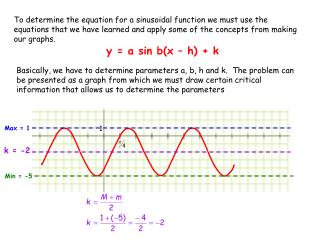DownloadDownload Presentationy = a sin b(x – h) + k

# y = a sin b(x – h) + k

Télécharger la présentation## y = a sin b(x – h) + k

- - - - - - - - - - - - - - - - - - - - - - - - - - - E N D - - - - - - - - - - - - - - - - - - - - - - - - - - -
##### Presentation Transcript

1. 1 To determine the equation for a sinusoidal function we must use the equations that we have learned and apply some of the concepts from making our graphs. y = a sin b(x – h) + k Basically, we have to determine parameters a, b, h and k. The problem can be presented as a graph from which we must draw certain critical information that allows us to determine the parameters Max = 1 k = -2 Min = -5

2. π 1 Period is the difference between consecutive maximums or consecutive minimums.

3. 3 1 Max = 1 Min = -5 The amplitude is the difference between the maximum and the minimum. We can use the fact that A = |a| to find parameter a.

4. 1 The phase shift (D) for the sin graph is the first equilibrium point before a maximum

5. 1 y = a sin b(x – h) + k k = -2 a = 3 b = 2